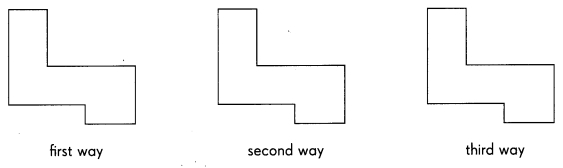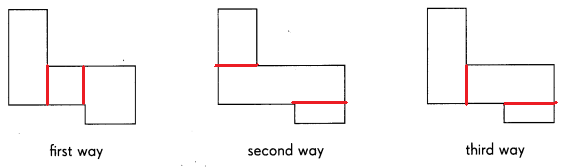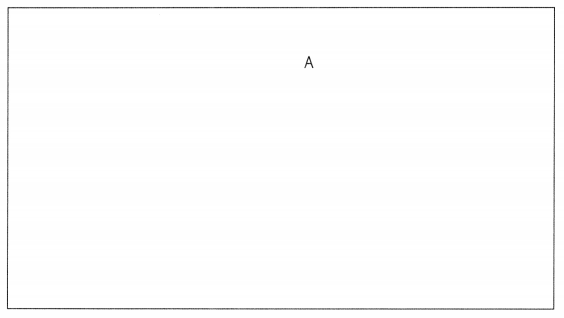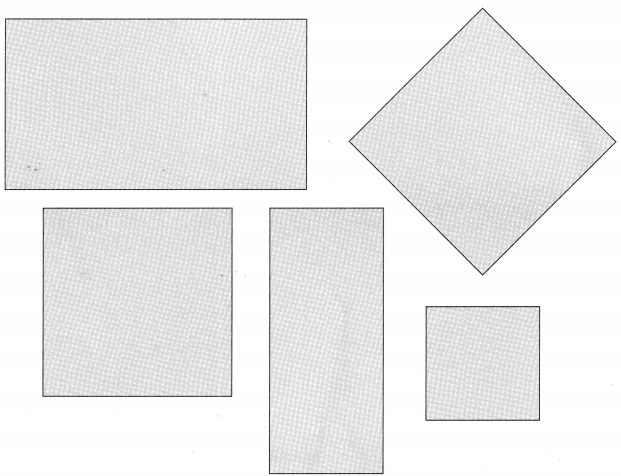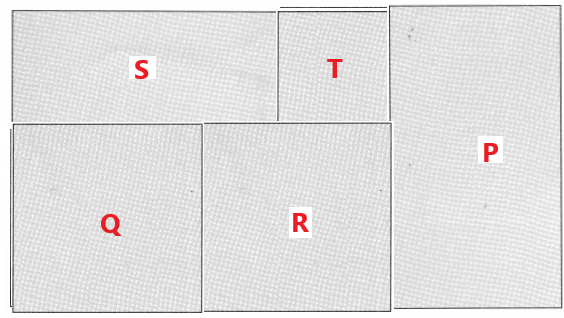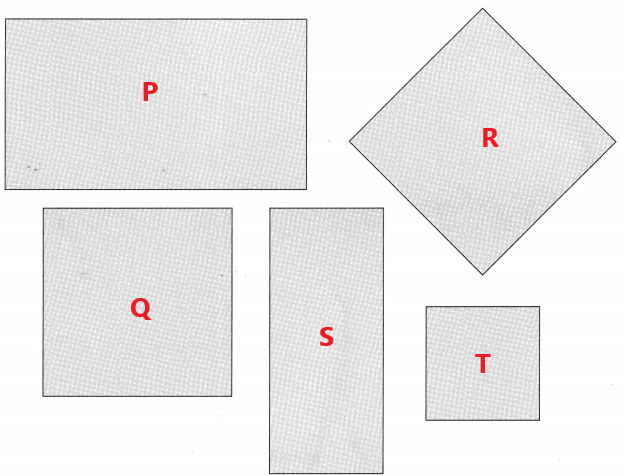# Math in Focus Grade 4 Chapter 11 Answer Key Squares and Rectangles

This handy Math in Focus Grade 4 Workbook Answer Key Chapter 11 Squares and Rectangles detailed solutions for the textbook questions.

## Math in Focus Grade 4 Chapter 11 Answer Key Squares and Rectangles

Math Journal

Figure ABCD is a rectangle. Complete each statement. Use the words in the box.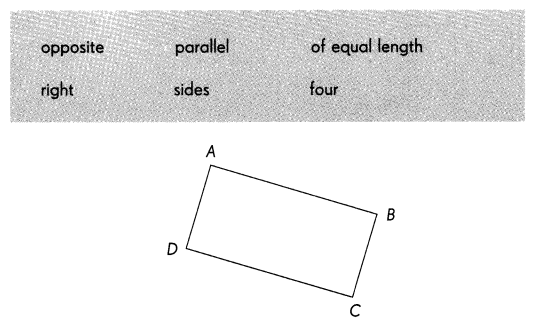Question 1.
A rectangle has ___________ ___________
A rectangle has four sides.

Question 2.
Its _______________ sides are ____________
Its opposite sides are parallel.

Question 3.
Its ____________ sides are ____________
Its opposite sides are of equal length.

Question 4.
It has ____________ ____________ angles.
It has four right angles.

Challenging Practice

Question 1.
The figure is made up of two squares, one with 10-inch sides and the other with 6-inch sides. Find QR.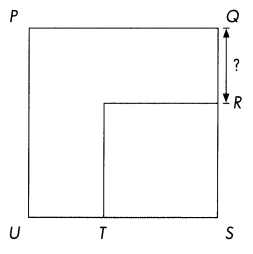QR = ___________ in.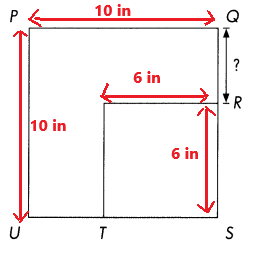In the above image we can observe QS = 10 inches and RS = 6 inches
QR = QS – RS
= 10 – 6
= 4 inches
QR = 4 inches

Question 2.
The figure is made up of three identical squares with 3-inch sides. Find the total length of $$\overline{B C}$$ and $$\overline{F G}$$ .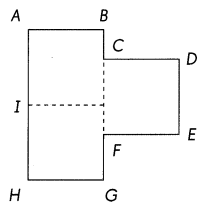BC + FG = ____________ in.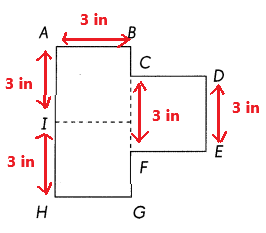BC + FG = AH – CF
= 6 –  3
= 3
BC + FG = 3 inches

Problem Solving

Question 1.
Look at the figure. What is the least number of squares that must be added to make a rectangle?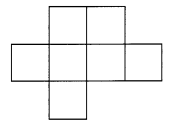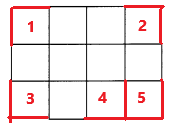To make rectangle we need to add 5 squares to the above figure.

Question 2.
Draw line segments to divide the figure into three rectangles in three ways.